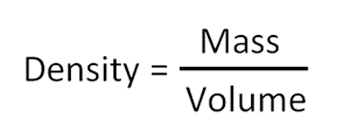Scientific Method
Vocabulary
Physics
Matter and Energy
Limitations
100

BONUS 300 points!

What are the three main steps of the Scientific Method, according to the book?

Observe, Hypothesize, and Experiment

100

Define energy as define in science.

The ability to do work

100

Define physics.

The study of interactions between matter and energy

100

Describe the difference between physical and chemical properties.

Physical: changing these properties does not change the type of matter.

Chemical: changing these DOES change the type of matter

100

Name 2 ways in which science is limited.

Scope, Assumptions, Bias, and Approximations

200

Who discovered that gravity acts on all objects equally, regardless of mass?

BONUS 200 points: Who incorrectly assumed gravity acts according to mass?

Galileo Galilei;

Aristotle

200

What's the difference between a law and a theory?

Laws: Theories that stand the test of time and have become accepted.

Theories: a hypothesis that passes the test of many experiments and has the support of other scientists.

200

BONUS 500 points!

Name 5 branches of physics.

Thermodynamics, Acoustics, Modern Dynamics, Classic Mechanics, Astrophysics, Optics, Electromagnetism, Nuclear Physics

200

Is density a physical or a chemical property?

Physical.

200

What books have answers to the questions of the soul?

The Bible and the Book of Mormon

300

What is "an artificial situation that more clearly shows how things happen."

An experiment

300

Define inertia

The tendency of matter to resist change.
300
How does physics relate to other fields of science?

Considered "the foundational science." All other fields of science depend on a proper understanding of physics.

300

What is the difference between mass and weight?

Mass tells the amount of inertia (or matter)

Weight describes the gravitational pull on an object

300

What is bias (in science)?

Human nature often leads one to see and believe what he wants to be true, not necessarily what is actually true.

400

What is the difference between a prediction and a hypothesis?

Prediction: Specific to an experiment

Hypothesis: Generalized guess about a principle

400

This vocabulary term literally means "does not follow"

Non sequitur

400

Rearrange the following equation to answer the question, "How much inertia does an object have?"m = d*v

400

___________ is an indirect measurement of an object's thermal energy.

Temperature

400

What example of bias did we talk about in class?

John Needham's "spontaneous generation" experiment, vs. Louis Pasteur's experiment.

500

What is the principle of causality?

The law of cause and effect. Events observed in the universe have rational causes; the same cause will always have the same effect.

500

Define equation.

A law of the universe expressed as a mathematical sentence.

A set of symbols summarizing a mathematical relationship between quantities.

500

Albert Einstein said, "The whole of science is nothing more than..."

a refinement in everyday thinking.

500

Give three examples of physical properties, and three examples of chemical properties

Physical: mass, weight, volume, density, temperature, state

Chemical: flammability, oxidation state, toxicity, reactivity, chemical bonding

500

What two reasons are given in the book of why we should study science?

1. The study of science leads us to glorify God.

2. Studying science can benefit man.

Click to zoom